This posting describes the process of decrypting ciphertext produced by a block cipher using a “padding oracle”. This idea – especially relating to the ASP.NET bug MS10-070 – has been documented before but I’m going to lay it out step-by-step with diagrams. I’m no cryptographer but I do find it interesting and walking it through in a way that makes sense to me will be useful for another day when I’ve forgotten it all! Hopefully it will be useful to someone else too. Note that I’m taking a general case here and the technique described below does not apply exactly to all of the cases when a padding oracle can be exploited. Maybe that will be another posting one day. That said, let’s get on with it.

The thing about a block cipher is that it requires the input to be a whole number of blocks with each block being a fixed size. When a plaintext message isn’t exactly divisible by the block size, the last block needs some padding to make it valid. The last byte of the last block tells you how many bytes of padding there are and each padding byte has that same value.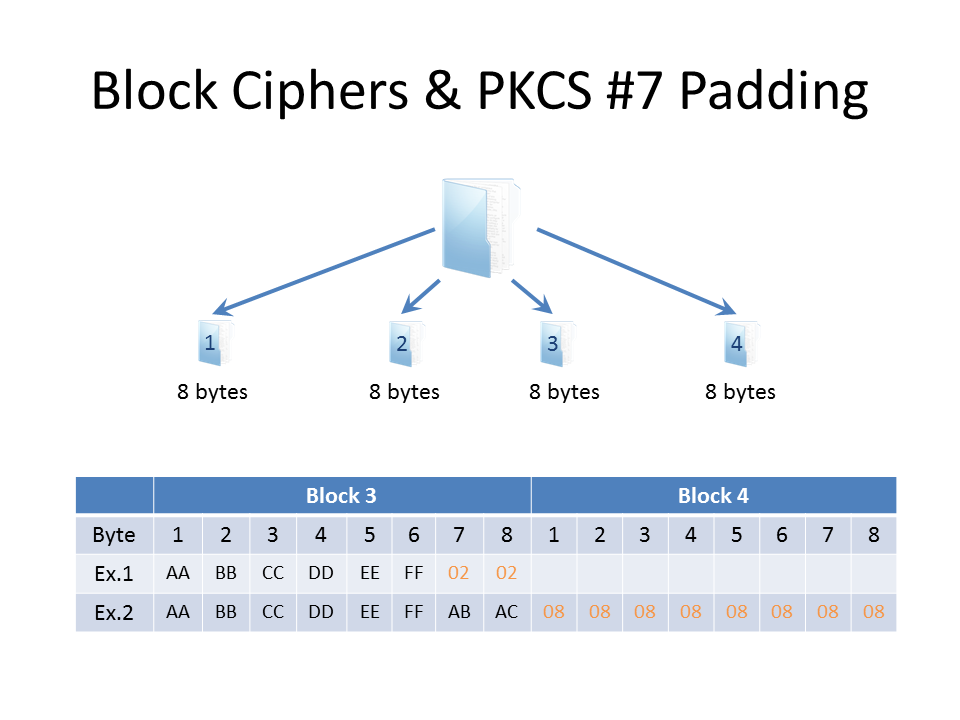In the diagram above we have a plaintext message broken up into blocks of 8 bytes – i.e. the cipher has a block size of 8 bytes. The last two blocks are set out in the table with two example rows. In the first example (Ex. 1) the message fits into three blocks with the third block ending with two bytes of padding (in orange). So each padding byte has a value of 2. In the second example (Ex.2) the message is exactly 24 bytes long (three blocks) and therefore another block is required for padding. Since the entire fourth block just contains padding, each of its bytes has a value of 8.

Encryption using CBC

When a block cipher is used in Cipher-Block-Chaining (CBC) mode, before a plaintext block is encrypted it is first XORed with the ciphertext of the previous block (as shown below). This creates an interdependence between the ciphertext blocks that cryptographers just love.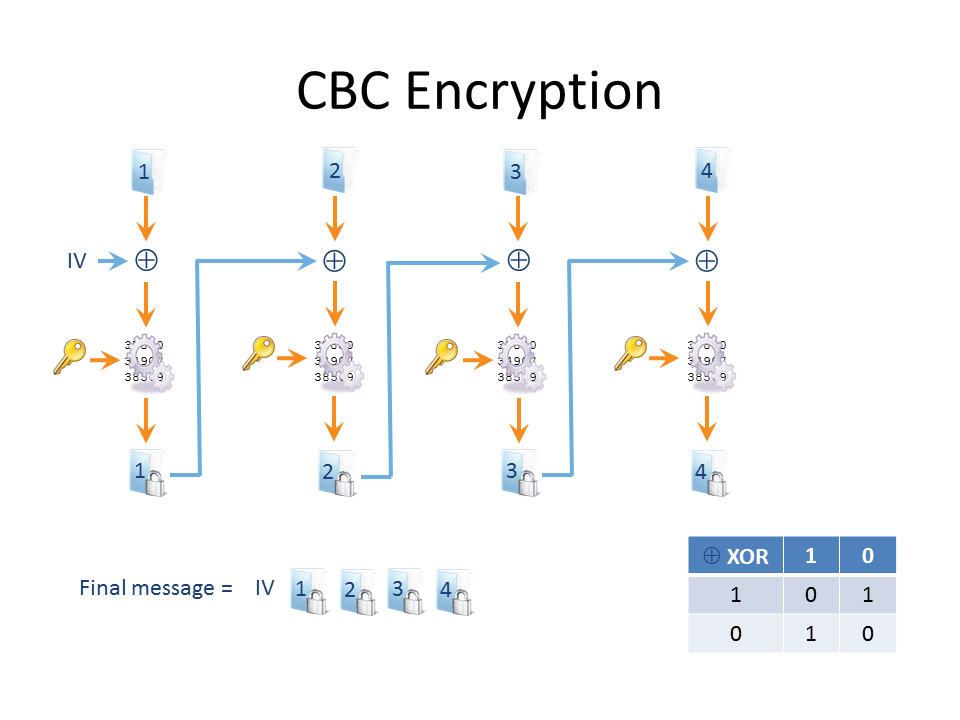The “truth table” for XOR is also shown: when both inputs are identical, XOR returns a zero; when both inputs are different, XOR returns a one. But what about the first block when there is no previous block? In this case, an initialisation vector (IV) can be used – it’s simply a random value (well, it doesn’t have to be random but it should be), exactly the same size as the block size. The IV ensures that the same plaintext message won’t produce the same ciphertext. Of course, for the message to be decrypted in full successfully, the IV must be known to the recipient somehow. If the recipient doesn’t know it by prior arrangement, it is simply sent along with the ciphertext message.

CBC Decryption and the Padding Oracle

The decryption process is shown below: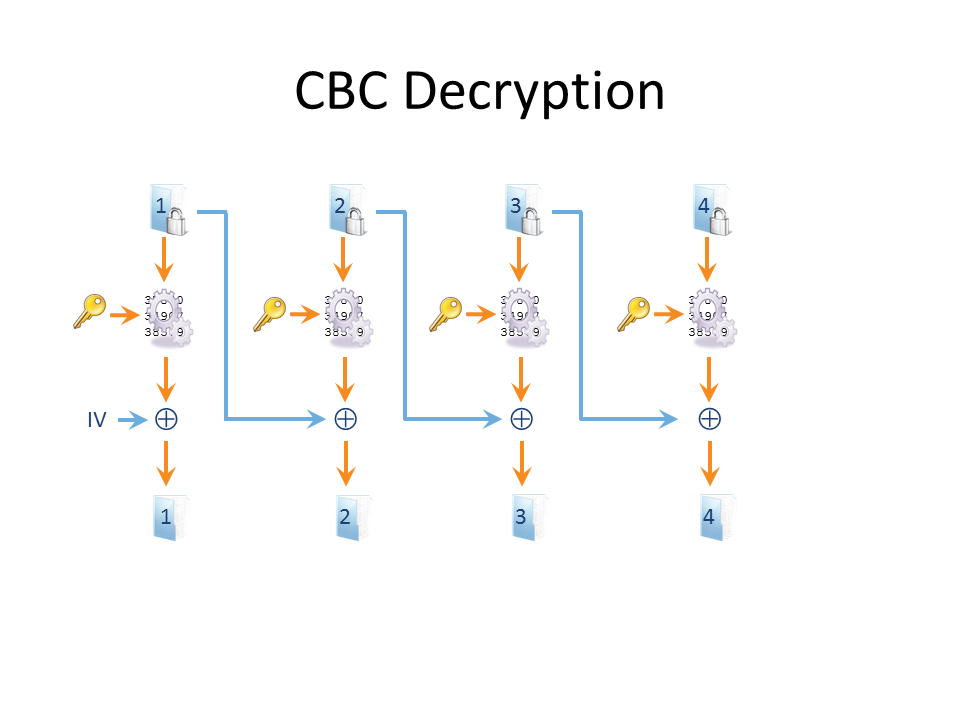When decrypted, the last block’s padding is checked so that the end of the plaintext is known. The last byte must be some number between 1 and the block size inclusive – and all the padding bytes must contain that same number. If not, something has gone wrong. In crypto circles an “oracle” is a database black box that leaks information so a “padding oracle” is an oracle that tells you whether or not the padding of a plaintext message is correct.

Using the Padding Oracle for Decryption

Let’s start our attack with the first block (you could also start with last block).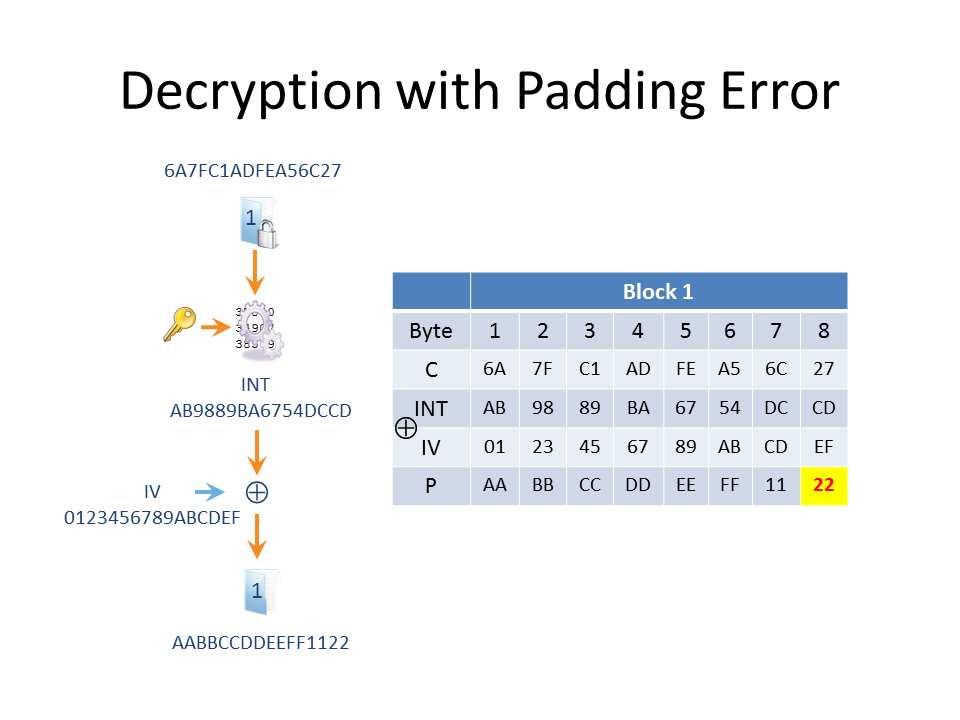We don’t know the Plaintext or the Key or the Intermediate bytes (that is, the bytes that drop out of the cipher, which then require XORing to produce the plaintext). But we do have control over the IV and the ciphertext that we send for decryption. If we send this single block as it stands (i.e. just the IV and the first block of ciphertext) to our “padding oracle”, we get a padding error returned as the last plaintext byte is not valid. There can’t be 22 bytes of padding in an 8-byte block cipher!

So how do we start guessing the plaintext? The attack starts by targeting the last plaintext byte (which we’ll denote as P8, the 8th plaintext byte) of the first block. Since we’re only sending this first block for decryption, the aim to is produce a P8 of 1, which is a valid padding byte.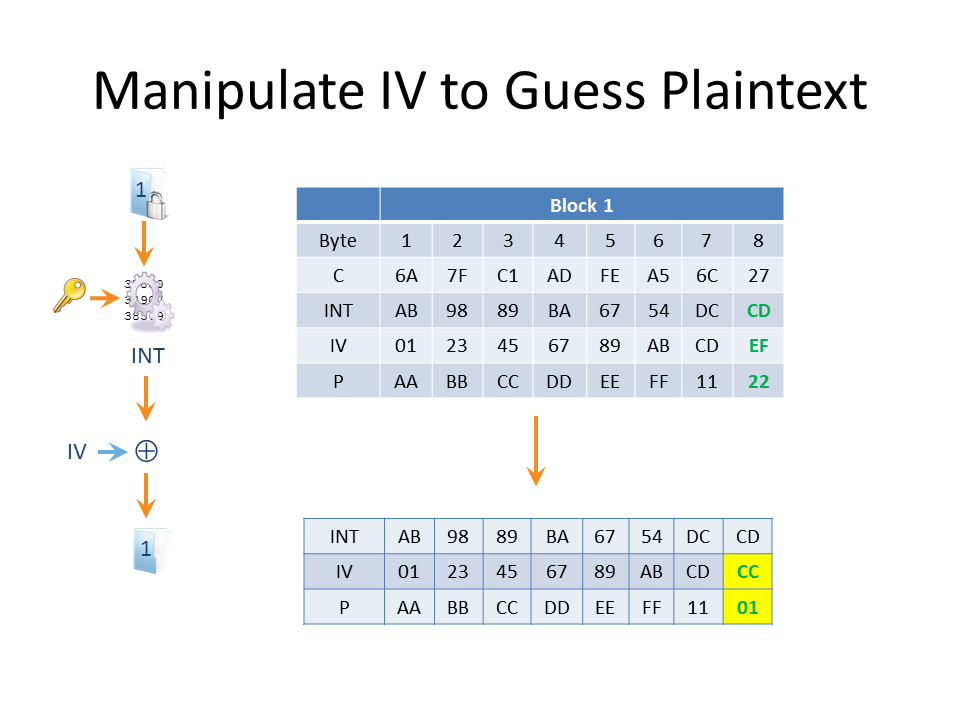Remember that P8 = INT8 XOR IV8 and currently that’s CD XOR EF = 22. Let’s guess that P8 = G (G for guess). We’ll now change the IV we send so that the last byte IV8 = IV8 XOR G XOR 1. The result is that the padding oracle won’t calculate the real P8 but P8 XOR G XOR 1. This is because we’ve effectively XORed G XOR 1 to both sides of P8 = INT8 XOR IV8. When the guess is correct and G = P8 the padding oracle calculates P8 XOR P8 XOR 1. Anything XORed with itself is 0 and anything XORed with 0 is unchanged (look back at the truth table) so P8 XOR P8 XOR 1 = 0 XOR 1 = 1. Thus when we guess correctly, the last byte will be a valid padding byte.

Let’s see what happens when we guess correctly in the above example. When we make a guess of G = 22, which really is the last byte of plaintext, we change IV8 to be EF XOR 22 XOR 1 = CC. When this is decrypted P8 = CD XOR CC = 1 and the padding oracle doesn’t complain. Of course the message is most likely gibberish as we’ve truncated the ciphertext to just the first seven bytes, and this might produce a different error, but the point is it’s not a padding error. When we guess wrong, we get a padding error; when we guess right, we get either no padding error or a different error message.

The Next Byte

Since the byte we’re targeting has 256 possible values, it will take no more than 256 guesses to figure out what the value of P8 is (of course, you could infer it after 255 failed guesses but it’s always nice to get positive confirmation!). We can better this if we know something about the plaintext byte, such as that it’s a printable character, in which case we can moderate our guesses. Once we know P8, we target the 7th byte in the same way – except that this time we want the final two bytes to be 2.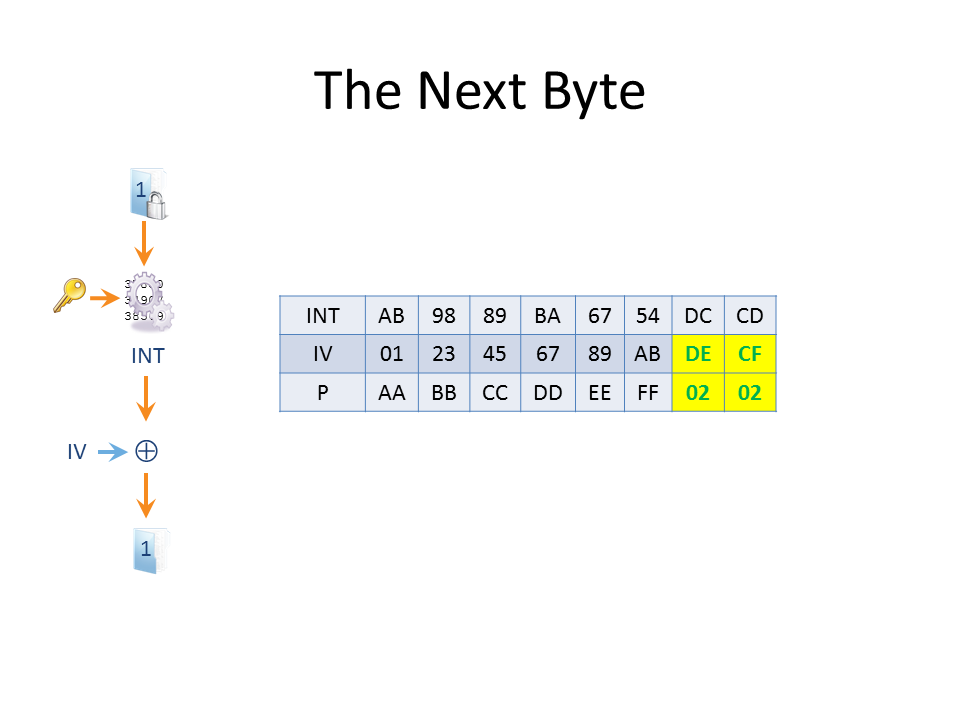First we fix IV8 so that P8 will always evaluate to 2. In the example above, we found that P8 = 22 and we know that IV8 = EF, thus INT8 = 22 XOR EF = CD. To make P8 = 2 we set IV8 = CD XOR 2 = CF. Now we change the IV so that the 7th byte (IV7) = IV7 XOR G XOR 2 where G is the guess for P7. When the guess is correct we’ll make P7 = 2 and the overall padding for the block will now be correct, as shown above. The correct guess of 11 creates an IV7 byte = CD XOR 11 XOR 2 = DE (where CD was the original value of IV7). When XORed with the corresponding intermediate byte INT7 we get P7 = DC XOR DE = 2, as required. Repeating this process will decrypt the first block of plaintext.

The Next Block

So what about the other blocks? To find the first plaintext block we manipulated the IV but the second block of plaintext doesn’t depend on the IV but on the first block of ciphertext, over which we also have control.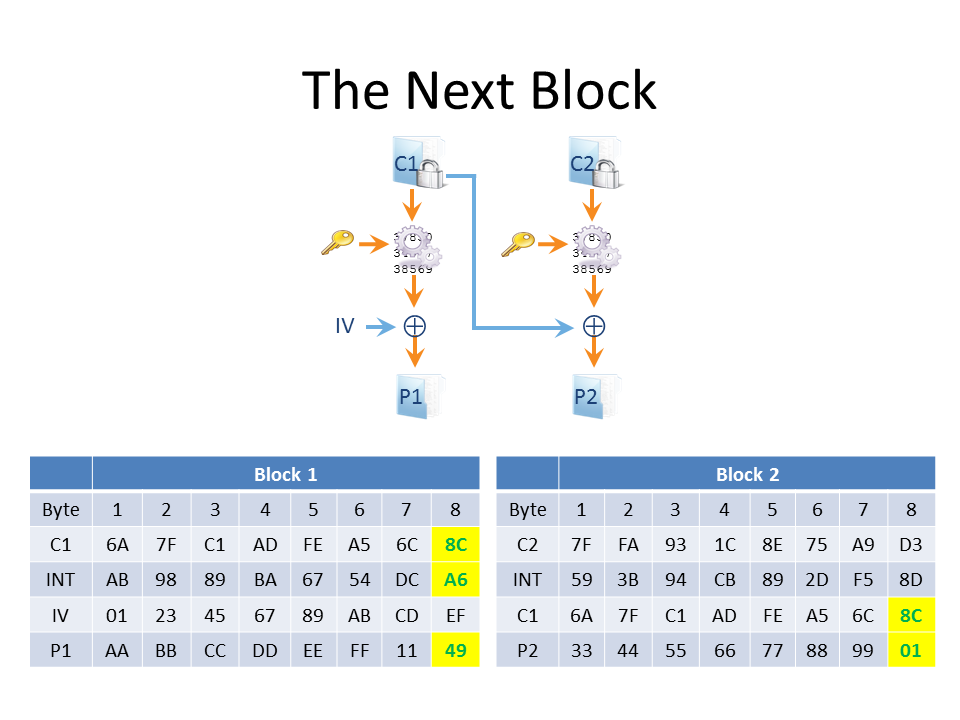To progress, then, we feed the padding oracle with the IV and the first two ciphertext blocks, and we simply use the same trick as before – but instead of manipulating the IV, we edit the first block of ciphertext. In the diagram above we’re targeting the last byte of block 2 by manipulating the last byte of ciphertext block 1. Again, what we’re aiming for at this stage is for the plaintext of the last byte of block 2 to evaluate to 1. When this happens the plaintext passes the padding check, but to achieve this we have corrupted the last byte of plaintext for block 1 (and the message is still truncated, remember). Again, though, it’s the absence of the padding error that we care about.

So that’s the general idea of how ciphertext encrypted under a block cipher can be decrypted when a padding oracle exists – and it all boils down to simple information leakage.